# Understanding and Automating Viewer Position Angles

Problem:

How do I change the viewing angles Psi, Alpha, and Theta from the viewer’s perspective? Also, please help me understand how these angles are defined.

Solution:

Below is a diagram from the 360 User’s Manual describing how Tecplot defines Psi (Ψ), Theta (θ), and Alpha (α).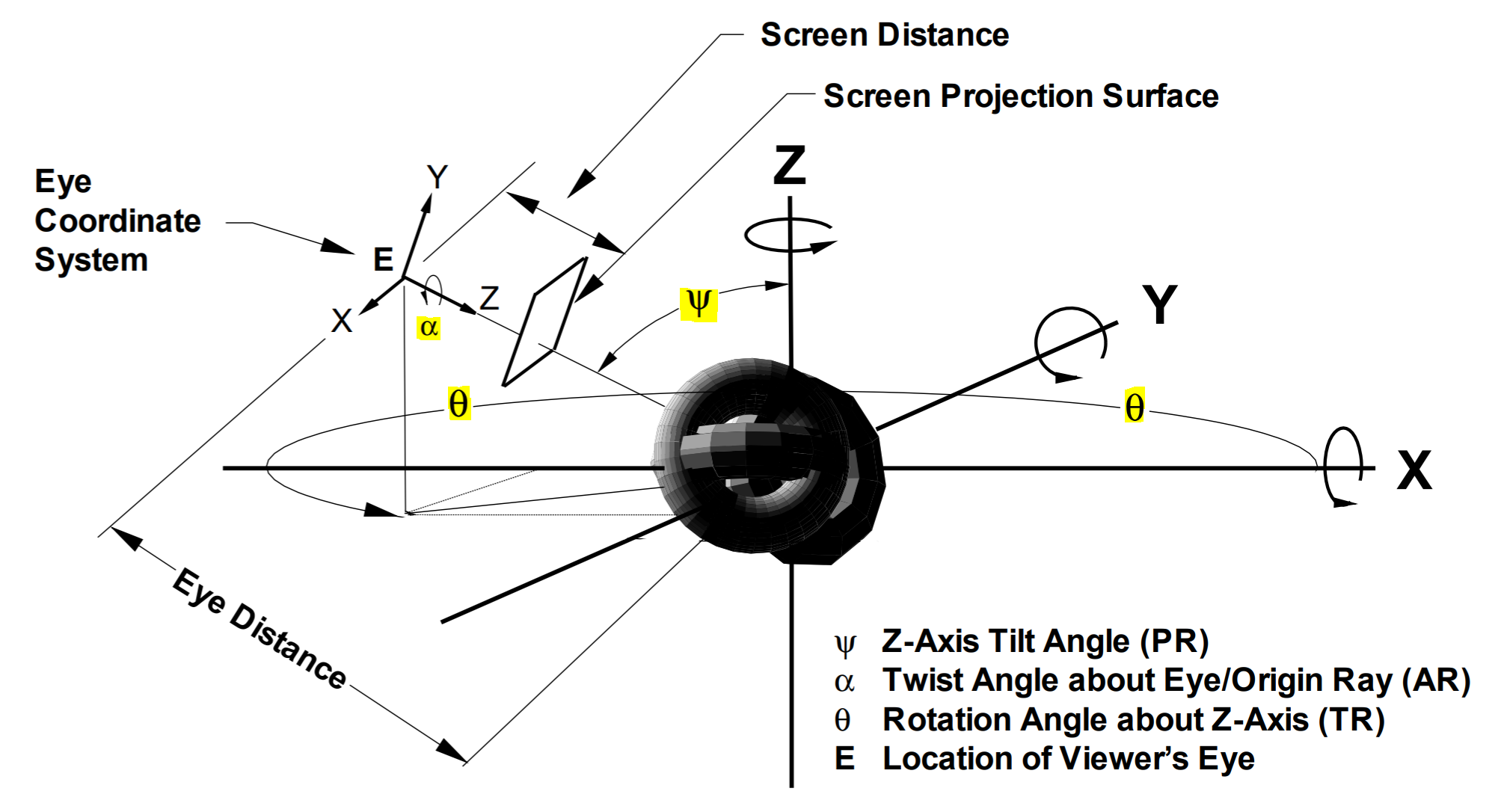Figure 1: For this KB article, we will focus on Ψ, θ, and α. For more information about this diagram, see the Rotate Dialog section of the User’s Manual.

The following exercise uses PyTecplot to change each of the three angles by 5 degrees and manifests the result after each step.

1)  Start with a 1x1x1 cube in the default viewing position in the first quadrant, see Figure 2.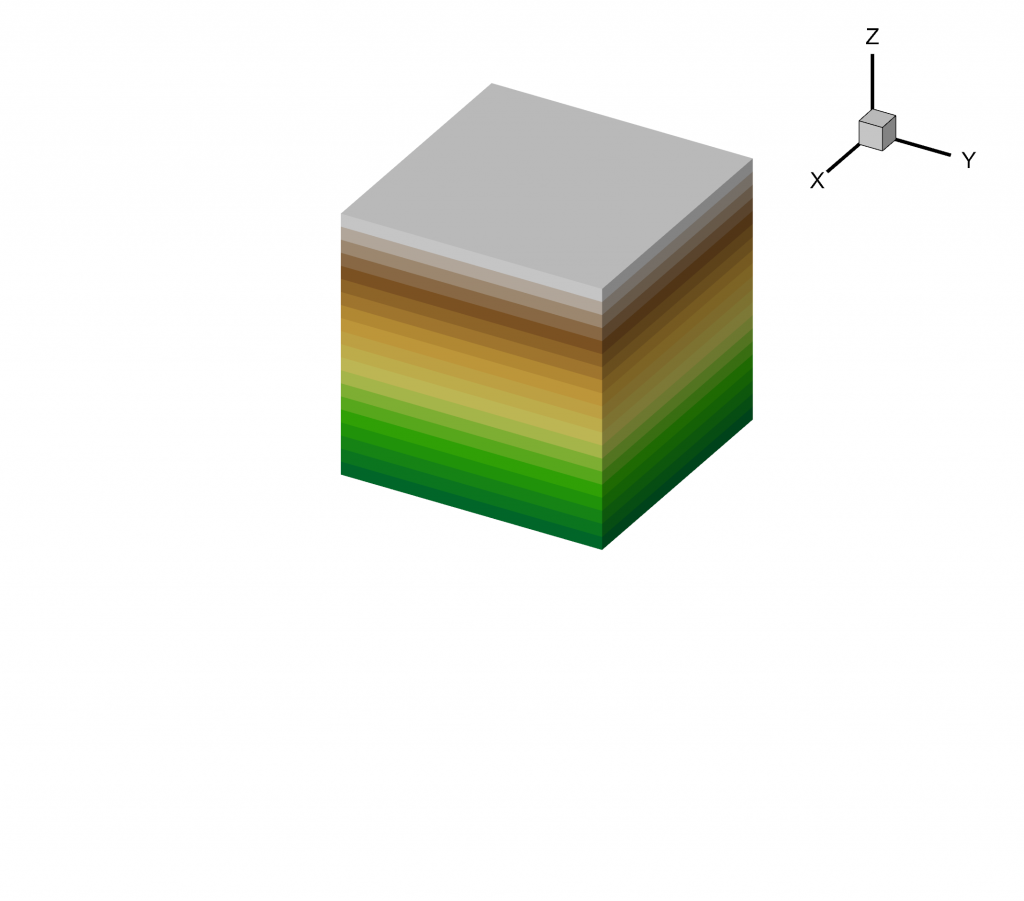Figure 2: This is the starting layout – a simple cube zone at the default 3D viewing position. Extra space is given for the Ψ, θ, and α angle transformations to come.

2)  Make sure the Tecplot work-space is accepting PyTecplot connections. Go to Scripting>PyTecplot Connections… and toggle-on Accept Connections.

3)  Run the following preliminary commands in a terminal to use PyTecplot (assuming PyTecplot is installed):

```C:\Users\user>python
>>> import tecplot as tp
>>> tp.session.connect()```

4)  To test changing the Ψ viewing angle by +5 degrees, run the following in the terminal. See the result in Figure 3.

`>>> plot.view.psi += 5.0`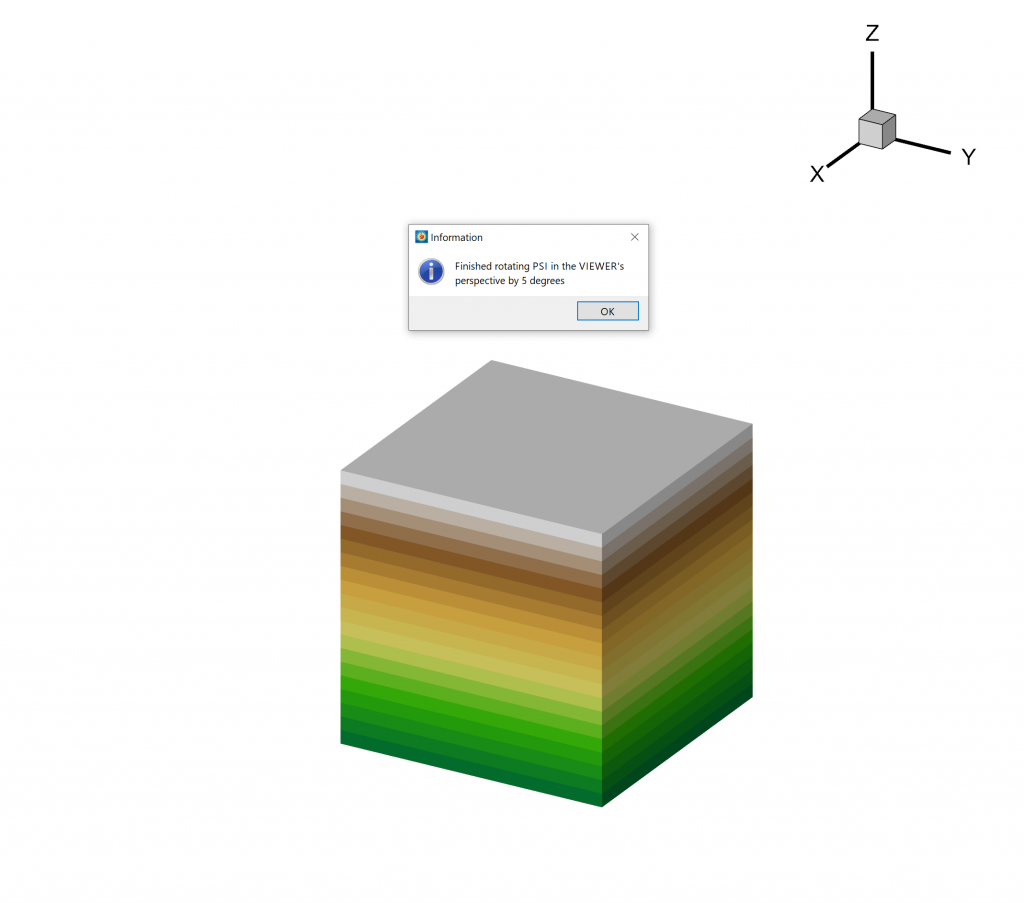Figure 3: Rotating Ψ by 5 degrees makes the camera look up (in this example starting with the default XYZ 3D view).

5)  To test changing the θ viewing angle by +5 degrees, run the following script. See the result in Figure 4.

`>>> plot.view.theta += 5.0`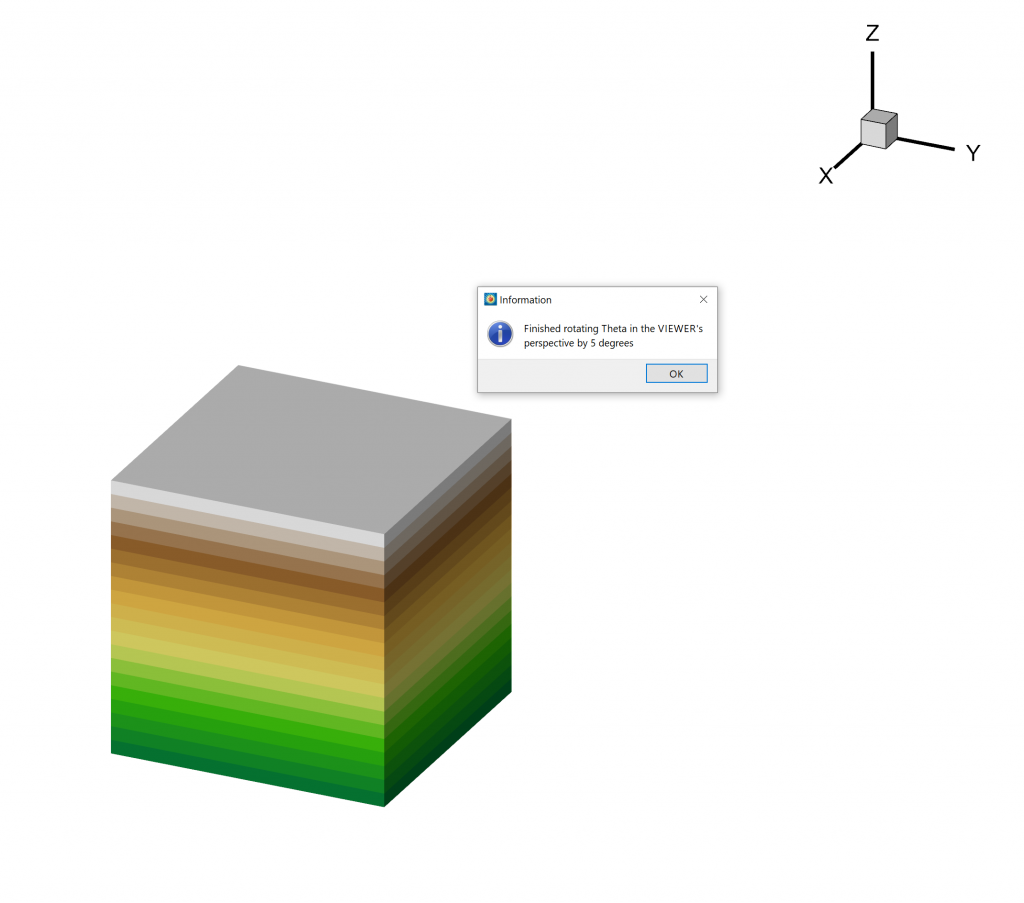Figure 4: Rotating θ by 5 degrees makes the camera look to the right in this example.

6)  To test changing the α angle by +5 degrees, run the following in the terminal. See the result in Figure 5.

`>>> plot.view.alpha += 5.0`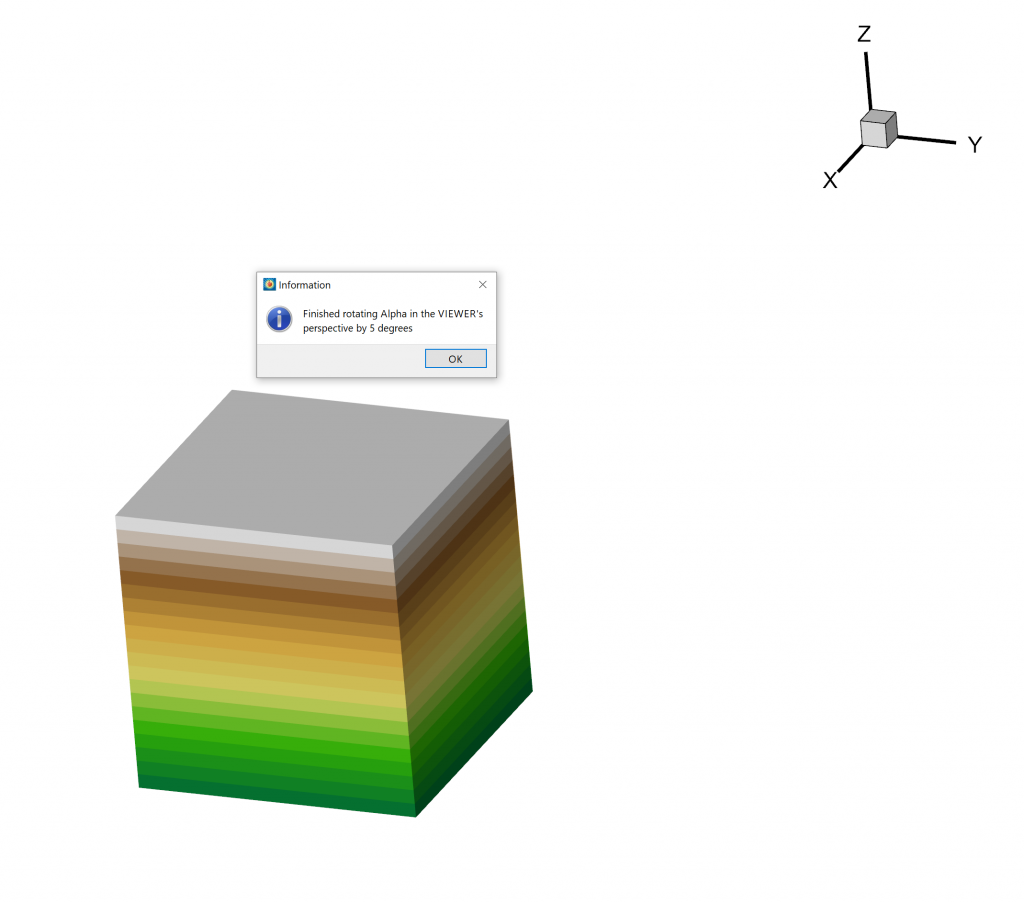Figure 5: Rotating alpha by 5 degrees makes the viewer’s perspective twist clockwise.